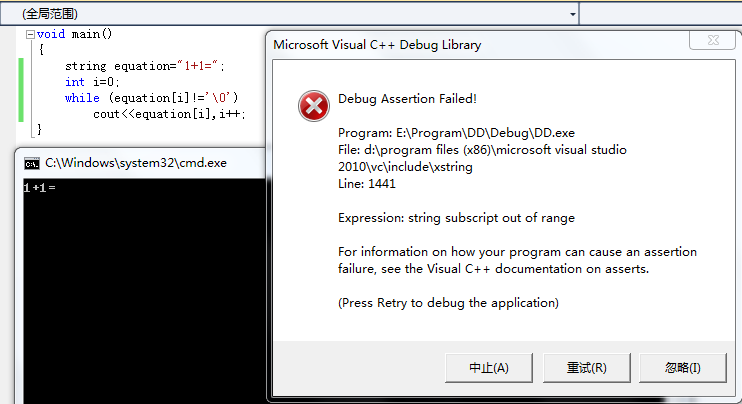string类在VS2010里和VC6.0里用法不一样？2个回答

`````` equation[i]
->
equation.c_str()[i]
``````3 年多之前 回复

while (equation[i] != 0)
->
while (i != equation.length())